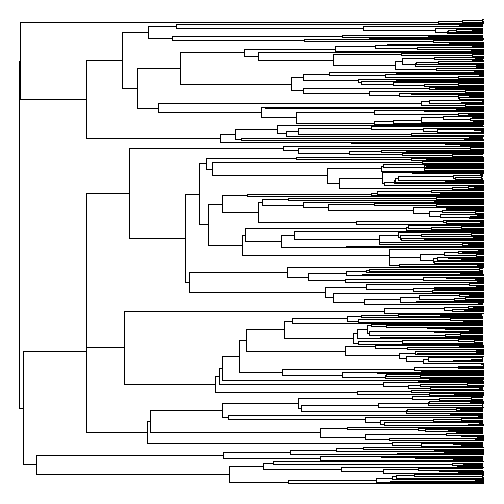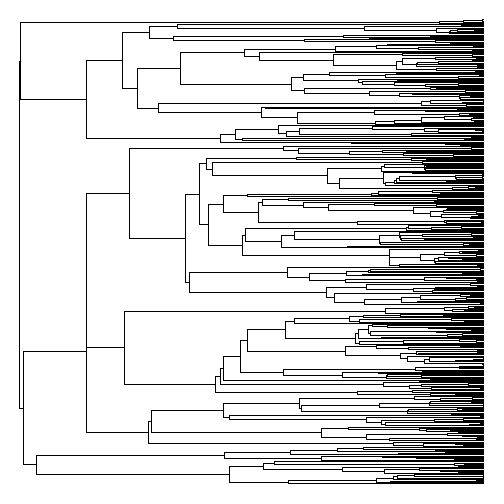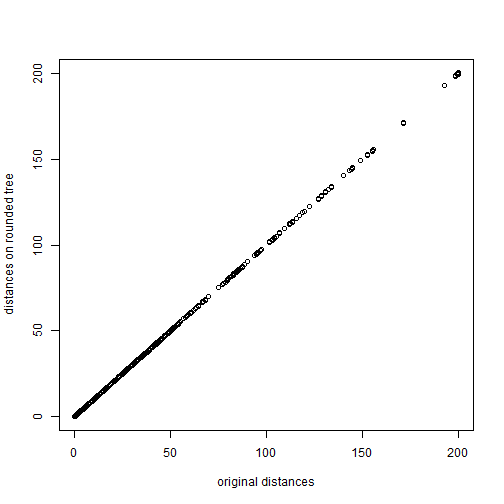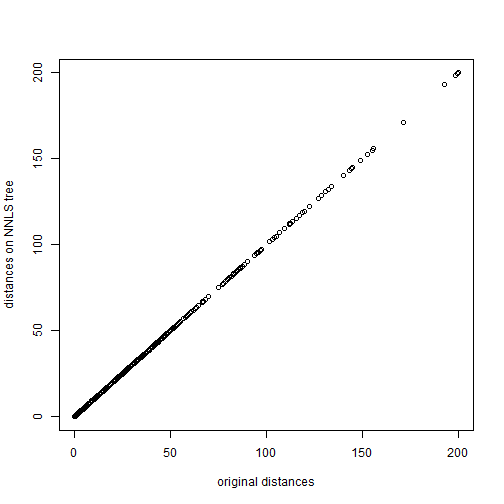## Tuesday, August 16, 2016

### "Fixing" an ultrametric tree whose edges are not ultrametric due to numerical precision / rounding

A R-sig-phylo subscriber posted the following question:

“I want to do some diversification pattern analyses with various R packages. I'm using a time-calibrated tree produced by PhyloBayes. Branch lengths are in millions of years and all taxa are extant, so the tree should be ultrametric. However, when I call `is.ultrametric`, it returns `FALSE`. Has anybody encountered something like this? Any ideas about what's going on/how to solve this?”

(Followed by some more details about check failures in different R analyses.)

My response was as follows:

“Since you are writing & reading trees to file, my guess is that it has to do with numerical precision - that is, the rounding of your edge lengths when they are written to file.

"Does your tree look ultrametric when plotted in R? If so, this is probably the case.

My recommendation is that you use phangorn to compute the non-negative least-squares edge lengths with the condition that the tree is ultrametric. This will give you the edge lengths that result in the distances between taxa with minimum sum of squared differences from the distances implied by your input tree, under the criterion that the resulting tree is ultrametric.”

Let's try this out using a simulated ultrametric tree that has had it's edges rounded - as if during writing to / reading from file:

``````library(phytools)
library(phangorn)
## simulate tree
tree<-otree<-pbtree(n=400,scale=100)
tree<-roundBranches(tree,1) ## round edges
## original tree ultrametric
is.ultrametric(otree)
``````
``````##  TRUE
``````
``````## rounded tree is not
is.ultrametric(tree)
``````
``````##  FALSE
``````
``````plotTree(tree,ftype="off",lwd=1) ## even though it looks ultrametric
````````````## compute the NNLS ultrametric tree
nnls<-nnls.tree(cophenetic(tree),tree,rooted=TRUE)
## check
is.ultrametric(nnls)
``````
``````##  TRUE
``````
``````plotTree(nnls,ftype="off",lwd=1)
``````Finally, we can compare our implied distances between the trees:

``````tips<-tree\$tip.label
plot(cophenetic(otree)[tips,tips],cophenetic(tree)[tips,tips],
xlab="original distances",ylab="distances on rounded tree")
````````````cor(as.vector(cophenetic(otree)[tips,tips]),
as.vector(cophenetic(tree)[tips,tips]))
``````
``````##  0.9999941
``````
``````plot(cophenetic(otree)[tips,tips],cophenetic(nnls)[tips,tips],
xlab="original distances",ylab="distances on NNLS tree")
````````````cor(as.vector(cophenetic(otree)[tips,tips]),
as.vector(cophenetic(nnls)[tips,tips]))
``````
``````##  0.9999995
``````

1.2.Hello Liam
Thanks for your post. However, when I tried to do
node.age(nnls)
I got this message:
Error in ages[n] = anc.age + phy\$edge.length[n] :
replacement has length zero
The problem seems to be that the edges of the nnls are in a different order than the original tree in edge:
> otree\$edge[1:3,]
[,1] [,2]
[1,] 401 402
[2,] 402 403
[3,] 403 404
> nnls\$edge[1:3,]
[,1] [,2]
[1,] 799 398
[2,] 799 399
[3,] 798 799

No idea why is this happening, but rotating the tree (ape::rotate(tree,length(tree\$tip.label)+1)
on the root solved the problem.

If there is a better way to solve the problem, will be great to know.
Thanks

Note: due to the very large amount of spam, all comments are now automatically submitted for moderation.